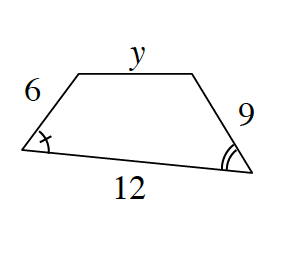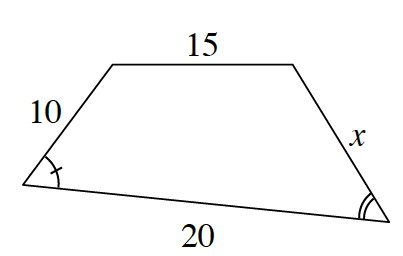### Home > CC3 > Chapter Ch7 > Lesson 7.2.2 > Problem7-54

7-54.

The figures below are similar. Use the information given about the lengths of the sides to solve for $x$ and $y$.Use proportions to solve for $x$ and $y$.

$\frac{6}{10}=\frac{\it y}{15}$

$10y=90$

$y=9$